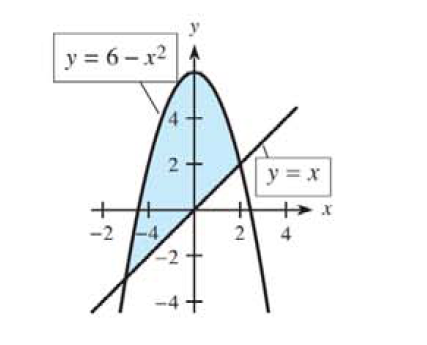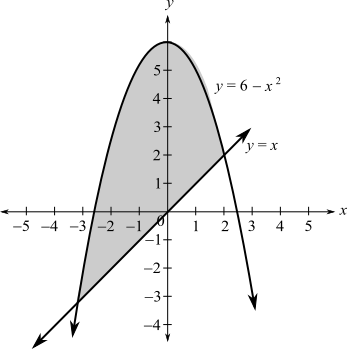Chapter 13.3, Problem 10E### Mathematical Applications for the ...

11th Edition
Ronald J. Harshbarger + 1 other
ISBN: 9781305108042

#### Solutions

Chapter
Section### Mathematical Applications for the ...

11th Edition
Ronald J. Harshbarger + 1 other
ISBN: 9781305108042
Textbook Problem

# For each shaded region in Problems 7-12, (a) find the points of intersection of the curves, (b) form the integral that represents the area of the shaded region, and (c) find the area of the shaded region.(a)

To determine

To calculate: The point of intersection of the curveExplanation

Given information:

The provided region is:

Formula used:

The steps for calculating the point of intersection of the curve are:

Step 1: Consider the equations in the curve.

Step 2: Solve the equations for any variable

Step 3: Substitute the value of the variable in any of the equation to calculate the value of other variable.

Calculation:

Consider the curve:

Consider the formula:

The equations are:

y=6x2

And

y=x

Substitute x for y in first equation to get:

x=6x2

Solve to get:

x2+x6=0(x

(b)

To determine

To calculate: The integral that represents the area of the shaded region.(c)

To determine

To calculate: The area of the region### Still sussing out bartleby?

Check out a sample textbook solution.

See a sample solution

#### The Solution to Your Study Problems

Bartleby provides explanations to thousands of textbook problems written by our experts, many with advanced degrees!

Get Started

#### Evaluate the expression sin Exercises 116. 230

Finite Mathematics and Applied Calculus (MindTap Course List)

#### The radius of convergence of is: 1 3 ∞

Study Guide for Stewart's Multivariable Calculus, 8th

#### Evaluate each expression. P(8,3)

College Algebra (MindTap Course List)